﻿ 一种改进的基于BiDirectional体制的MISO-SAR系统
«上一篇文章快速检索 高级检索

 雷达学报2015, Vol. 4Issue (5): 527-537  DOI: 10.12000/JR150220

### 引用本文 [复制中英文]

[复制中文]
Jiang Hai, Song Hong-jun. Improved MISO-SAR System Based on BiDirectional Imaging[J]. Journal of Radars, 2015, 4(5): xx–xx. DOI: 10.12000/JR15022.
[复制英文]

### 文章历史,Improved MISO-SAR System Based on BiDirectional Imaging,Institute of Electronics, Chinese Academy of Sciences, Beijing 100190, China;
University of Chinese Academy of Sciences, Beijing, 100049, China
Abstract: In 2012, the German Aerospace Center (DLR.) proposed a BiDirectional mode that can achieve several seconds of repeated time lags by single star and single flight. Its basic principle includes the generation of a double-beam antenna pattern by electronic beam steering and simultaneous emission of two pulses that irradiate the front and back imaging area. The two pulses, which are simultaneously received will be separated by band-pass filtering in the Doppler domain and imaged, respectively. This paper presents an improved Multi Input Single Output (MISO)-SAR system based on the BiDirectional mode which converts the traditional simultaneous dual beam emitting and receiving into time-division emitting and simultaneous receiving, respectively. This results in an improved emitting antenna pattern owning to the suppression of the Azimuth Ambiguity to Signal Ratio (AASR). The current paper describes the spectrum separation effects, AASR analysis, and the system design process. Therefore, to confirm effectiveness, point target 1-D and 2-D simulation results are compared before and after the improvement. Furthermore, the BiDirectional and other short-term repeated SAR modes are compared.
Key words: BiDirectional SAR     Multi Input Single Output (MISO)     Grating lobe     Azimuth Ambiguity to Signal Ratio (AASR)
1 引言

2 改进的MISO-SAR系统 2.1 相控阵天线方向图

 $G(\theta ) = {G_{\rm{e}}}(\theta ) \cdot \left| {{1 \over K}\sum\limits_{k = 0}^{K - 1} {{C_k},T \cdot \exp \left( {{\rm{j}}{{2\pi k} \over \lambda }{L_{{\rm{ae}}}}\sin \theta } \right)} } \right|$ (1)

 ${G_{\rm{e}}}(\theta ) = {G_0} \cdot \left| {{\mathop{\rm sinc}\nolimits} \left( {\frac{{{L_{{\rm{ae}}}}}}{\lambda }\sin \theta } \right)} \right|$ (2)
Ge(θ)表示天线阵元的方向图，K为T/R组件个数，Lae为天线阵元长度，在天线扫描时为避免栅瓣影响，应满足：
 ${L_{{\rm{ae}}}} \le \frac{\lambda }{{1 + \left| {\sin {\theta _{s,\max }}} \ \ \ \right|}}$ (3)
θs,max是天线最大扫描角度，以德国Terra SAR-X卫星为例[15, 16]，其扫描角度范围为±0.75°，工作频率为9.65 GHz，对应工作波长为0.0311 m，相控阵天线由384个天线单元组成，排布成12(方位向)×32(距离向)的阵列，天线总长度为4.8 m×0.75 m，对应的单元间距为0.4 m×0.022 m。天线主瓣和第1栅瓣的间隔角度可根据式(2)中的相位系数计算得出，如式(4)所示：
 $\Delta \theta = \arcsin \frac{\lambda }{{{L_{{\rm{ae}}}}}}$ (4)图 1 TerraSAR-X天线方向图仿真(扫描角2.2°) Fig.1 Simulation diagram of TerraSAR-X antenna pattern (steering angle 2.2°)
2.2 改进的几何关系图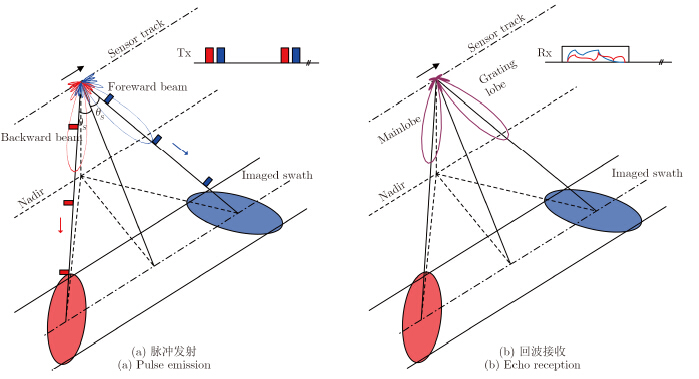图 2 改进的MISO-SAR体制几何关系图 Fig.2 Improved imaging geometry of MISO-SAR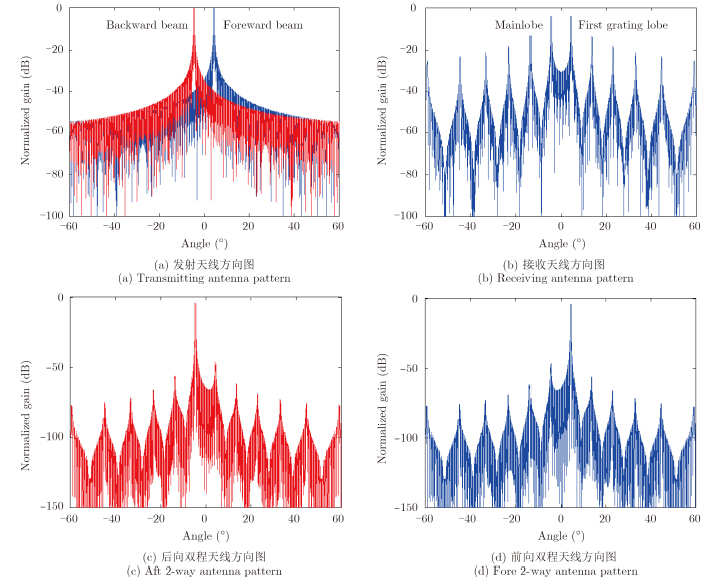图 3 MISO-SAR相控阵天线方向图 Fig.3 MISO-SAR phased array antenna pattern
2.3 频谱分离效果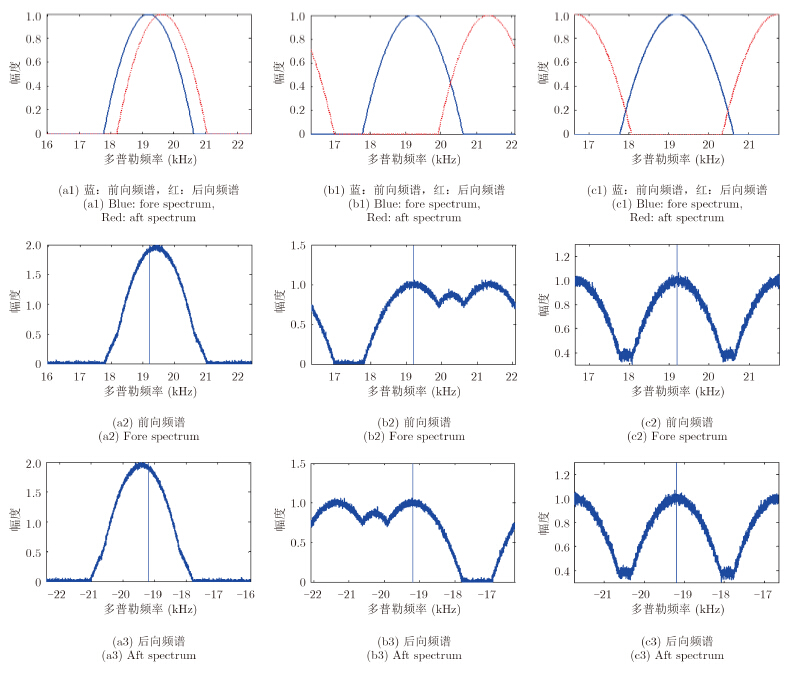图 4 不同PRF取值下前后向频谱分离效果 Fig.4 The effects of spectrum separation under different PRFs

3 AASR分析和系统设计 3.1 AASR分析

 ${\rm{AAS}}{{\rm{R}}_{\rm{b}}} = {{\sum\limits_{k \ne 0} {\smallint _{ - {B_{\rm{a}}}{\rm{/}}2 + {f_{{\rm{dc}},{\rm{b}}}}}^{{B_{\rm{a}}}{\rm{/}}2 + {f_{{\rm{dc}},{\rm{b}}}}}} \left[ {{G_{{\rm{t}},{\rm{b}}}}(f{_{\rm{a}}} + k \cdot {\rm{PRF}}) + {G_{{\rm{t}},{\rm{f}}}}(f{_{\rm{a}}} + k \cdot {\rm{PRF}})} \right] \cdot {G_{\rm{r}}}(f{_{\rm{a}}} + k \cdot {\rm{PRF}}){\rm{d}}{f_{_{\rm{a}}}}} \over {{\rm{ }}\smallint _{ - {B_{\rm{a}}}/2 + {f_{{\rm{dc}},{\rm{b}}}}}^{{B_{\rm{a}}}/2 + {f_{{\rm{dc}},{\rm{b}}}}}\left[ {{G_{{\rm{t}},{\rm{b}}}}({f_{\rm{a}}}) + {G_{{\rm{t}},{\rm{f}}}}({f_{\rm{a}}})} \right] \cdot {G_{\rm{r}}}({f_{\rm{a}}}){\rm{d}}{f_{\rm{a}}}}}$ (5)
 ${\rm{AAS}}{{\rm{R}}_{\rm{f}}} = {{\sum\limits_{k \ne 0} {\smallint _{ - {B_{\rm{a}}}{\rm{/}}2 + {f_{{\rm{dc}},{\rm{f}}}}}^{{B_{\rm{a}}}{\rm{/}}2 + {f_{{\rm{dc}},{\rm{f}}}}}} \left[ {{G_{{\rm{t}},{\rm{b}}}}({f_{\rm{a}}} + k \cdot {\rm{PRF}}) + {G_{{\rm{t}},{\rm{f}}}}({f_{\rm{a}}} + k \cdot {\rm{PRF}})} \right] \cdot {G_{\rm{r}}}({f_{\rm{a}}} + k \cdot {\rm{PRF}}){\rm{d}}{f_{\rm{a}}}} \over {\smallint _{ - {B_{\rm{a}}}{\rm{/}}2 + {f_{{\rm{dc}},{\rm{f}}}}}^{{B_{\rm{a}}}{\rm{/}}2 + {f_{{\rm{dc}},{\rm{f}}}}}\left[ {{G_{{\rm{t}},{\rm{b}}}}({f_{\rm{a}}}) + {G_{{\rm{t}},{\rm{f}}}}({f_{\rm{a}}})} \right] \cdot {G_{\rm{r}}}({f_{\rm{a}}}){\rm{d}}{f_{\rm{a}}}{\rm{ }}}}{\rm{ }}$ (6)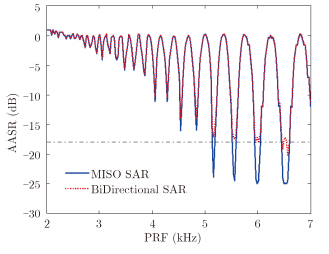图 5 MISO-SAR和BiDirectional模式下的AASR与PRF关系图 Fig.5 AASRs of BiDirectional and MISO-SAR modes under different PRFs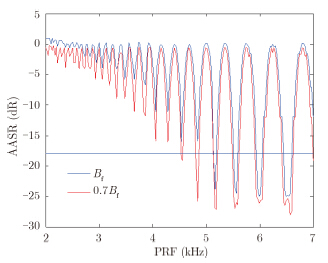图 6 MISO-SAR模式不同多普勒处理带宽下AASR与PRF关系图 Fig.6 MISO-SAR AASRs of different processed Doppler band under different PRFs
3.2 系统设计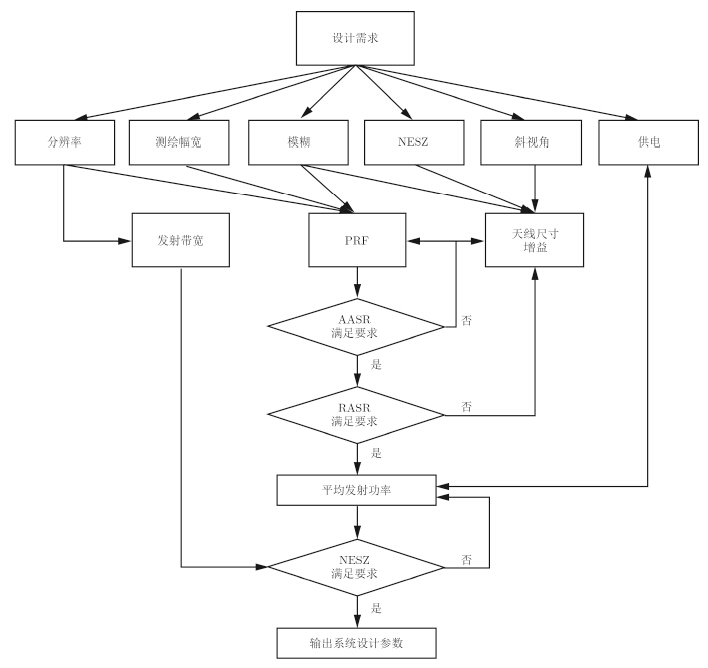图 7 基于BiDirectional体制的MISO-SAR系统设计流程 Fig.7 System design process of MISO-SAR mode based on BiDirectional mode
4 原始数据处理和仿真表 1 MISO-SAR仿真参数 Tab. 1 Simulation parameters of MISO-SAR mode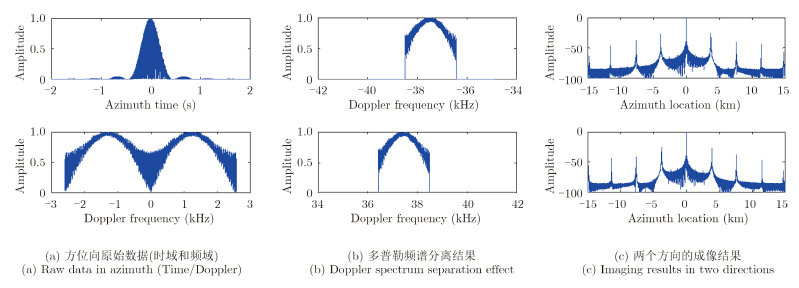图 8 PRF选择5120 Hz时MISO-SAR体制下仿真结果 Fig.8 Simulation results of MISO-SAR mode,PRF=5120 Hz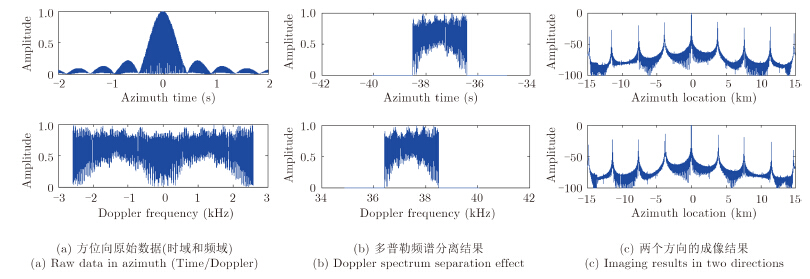图 9 PRF选择5120 Hz时传统BiDirectional体制下仿真结果 Fig.9 Simulation results of traditional BiDirectional mode,PRF=5120 Hz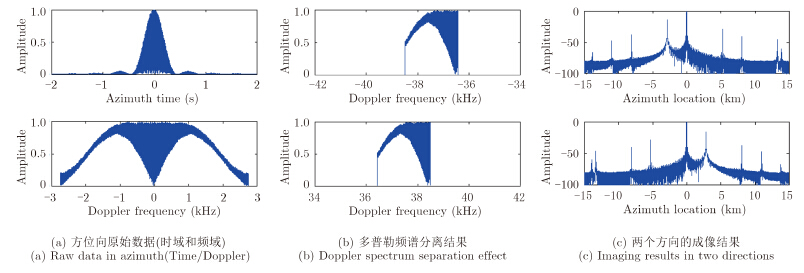图 10 PRF选择5490 Hz时MISO-SAR体制下仿真结果 Fig.10 Simulation results of MISO-SAR mode,PRF=5490 Hz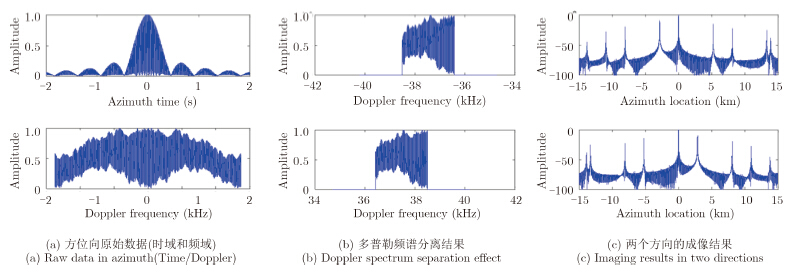图 11 PRF选择5490 Hz时传统BiDirectional体制下仿真结果 Fig.11 Simulation results of traditional BiDirectional mode,PRF=5490 Hz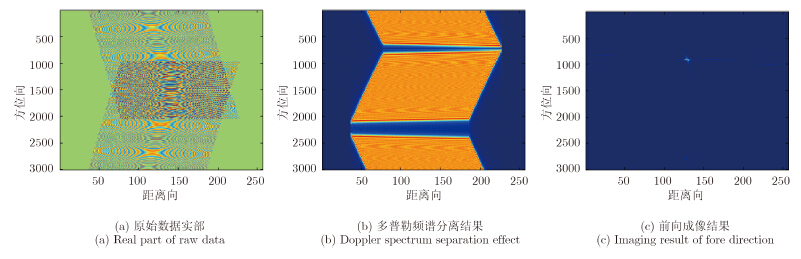图 12 PRF选择5120 Hz时BiDirectional体制下2维仿真结果 Fig.12 2-D simulation results of BiDirectional mode,PRF=5120 Hz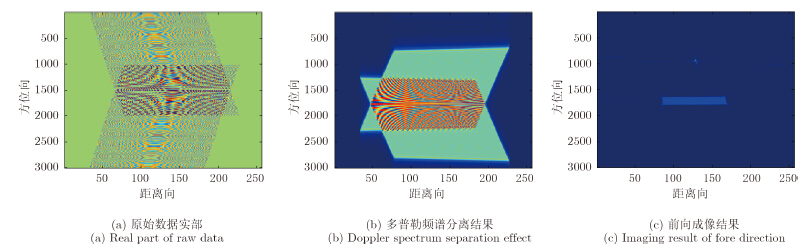图 13 PRF选择5490 Hz时BiDirectional体制下2维仿真结果 Fig.13 2-D simulation results of BiDirectional mode,PRF=5490 Hz
5 与其它单星重访体制的对比

5.1 条带多视

 ${t_{{\rm{lag,m}}}} = \frac{{{r_0} \cdot \Delta {\theta _{3\,{\rm{dB}}}}}}{{{V_{\rm{g}}}}} \cdot \left( {1 - \frac{1}{{{n_L}}}} \right)$ (7)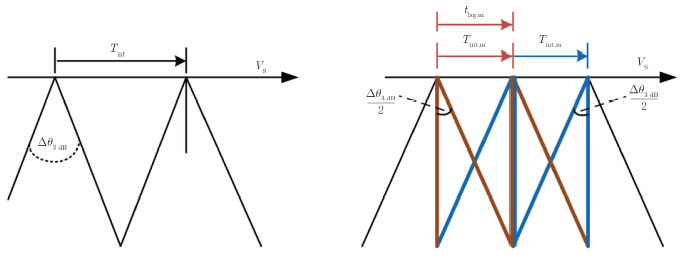图 14 条带单视和条带多视 Fig.14 Sing-look stripmap and 2-look stripmap acquisition

 ${t_{{\rm{max}}}} = \frac{{{r_0} \cdot \Delta {\theta _{3\,{\rm{dB}}}}}}{{{V_{\rm{g}}}}} = {T_{{\mathop{\rm int}} }}$ (8)

5.2 顺次前/后斜视条带

 ${t_{{\rm{lag}},{\rm{s}}}} = \frac{{{r_0} \cdot ({\psi _{{\rm{fore}}}} - {\psi _{{\rm{aft}}}} \ )}}{{{V_{\rm{g}}}}}$ (9)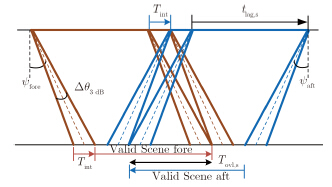图 15 顺次前/后斜视条带法 Fig.15 Sequential fore/aft stripmap acquisition

 ${T_{{\rm{ovl}},{\rm{s}}}} = \frac{{{r_0} \cdot ({\psi _{{\rm{fore}}}} - {\psi _{{\rm{aft}}}} - \Delta {\theta _{3\,{\rm{dB}}}} \ )}}{{{V_{\rm{g}}}}}$ (10)

5.3 绳针式前/后斜视条带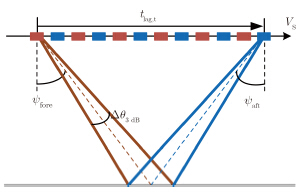图 16 绳针式前/后斜视条带法 Fig.16 Toggling fore/aft stripmap acquisition
5.4 几种重访体制的对比表 2 几种短时重访SAR体制对比 Tab. 2 Comparison of some short-term repetition SAR modes

6 总结

  Frasier S J and Camps A J. Dual-beam interferometry for ocean surface current vector mapping[J]. IEEE Transactions on Geoscience and Remote Sensing, 2001, 39(2): 401-414.(2)  D’Aria D, De Zan F, Giudici D, et al.. Burst-mode SARs for wide-swath surveys[J]. Canadian Journal of Remote Sensing, 2007, 33(1): 27-38.(1)  Xu Wei and Deng Yun-kai. Investigation on electronic azimuth beam steering in the spaceborne SAR imaging modes[J]. Journal of Electromagnetic Waves and Applications, 2011, 25: 2076-2088.(1)  Baumgartner S V and Krieger G. Large along-track baseline SAR-GMTI: first results with the TerraSAR-X/TanDEM-X satellite constellation[C]. IEEE Geoscience and Remote Sensing Symposium, Vancouver, Canada, 2011: 1319-1322.(1)  Mittermayer J, Wollstadt S, Baumgartner S, et al.. Approach to velocity and acceleration measurement in the Bi-Directional SAR imaging mode[C]. IEEE Geoscience and Remote Sensing Symposium, Munich, Germany, 2012: 5618-5621.(1)  Mittermayer J, Wollstadt S, Prats-Iraola P, et al.. Bidirectional SAR imaging mode[J]. IEEE Transactions on Geoscience and Remote Sensing, 2013, 51(1): 601-614.(3)  Mittermayer J, Schättler B, and Younis M. TerraSAR-X commissioning phase execution summary[J]. IEEE Transactions on Geoscience and Remote Sensing, 2010, 48(2): 649-659.(1)  Suchandt S, Runge H, Breit H, et al.. Automatic extraction of traffic flows using TerraSAR-X alongtrack interferometry[J]. IEEE Transactions on Geoscience and Remote Sensing, 2010, 48(2): 807-819.(1)  Hueso Gonzalez J, Bachmann M, and Hofmann H. TanDEM-X commissioning phase status[C]. IEEE Geoscience and Remote Sensing Symposium, Honolulu, Hawaii, 2010: 2633-2635.(1)  Mittermayer J, Younis M, Metzig R, et al.. TerraSAR-X system performance characterization and verification[J]. IEEE Transactions on Geoscience and Remote Sensing, 2010, 48(2): 660-676.(1)  Mittermayer J and Runge H. Conceptual studies for exploiting the TerraSAR-X dual receive antenna[C]. IEEE Geoscience and Remote Sensing Symposium, Toulouse, France, 2003: 2140-2142.(1)  Romeiser R, Suchandt S, Runge H, et al.. First analysis of TerraSAR-X along-track InSAR-derived current fields[J]. IEEE Transactions on Geoscience and Remote Sensing, 2010, 48(2): 820-829.(1)  Krieger G, Moreira A, Fiedler H, et al.. TanDEM-X: a satellite formation for high resolution SAR interferometry[J]. IEEE Transactions on Geoscience and Remote Sensing, 2007, 45(11): 3317-3341.(1)  Bamler R and Eineder M. Accuracy of differential shift estimation by correlation and split-bandwidth interferometry for wideband and delta-k SAR systems[J]. IEEE Geoscience and Remote Sensing Letters, 2005, 2(2): 151-155.(2)  Stangl M, Werninghaus R, and Zahn R. The TerraSAR-X active phased array antenna[C]. IEEE International Symposium on Phased Array Systems and Technology, Boston, USA, 2003: 70-75.(1)  Suess M, Riegger S, Pitz W, et al.. TERRASAR-X-Design and performance[C]. Proceedings of EUSAR, Koln, Germany, 2002: 133-136.(1)  Ouchi K. On the multilook images of moving targets by synthetic aperture radars[J]. IEEE Transactions on Antennas and Propagation, 1985, 33(8): 823-827.(1)  Prats P, Marotti L, Wollstadt S, et al.. Investigations on tops interferometry with TerraSAR-X[C]. IEEE Geoscience and Remote Sensing Symposium, Honolulu, Hawaii, 2010: 2629-2632.(1)